International
Tables for
Crystallography
Volume B
Reciprocal space
Edited by U. Shmueli

International Tables for Crystallography (2006). Vol. B. ch. 2.4, pp. 266-267   | 1 | 2 |

## Section 2.4.3.2. Violation of Friedel's law

M. Vijayana* and S. Ramaseshanb

aMolecular Biophysics Unit, Indian Institute of Science, Bangalore 560 012, India, and bRaman Research Institute, Bangalore 560 080, India
Correspondence e-mail:  mv@mbu.iisc.ernet.in

#### 2.4.3.2. Violation of Friedel's law

| top | pdf |

Consider a structure containing N atoms of which P are normal atoms and the remaining Q anomalous scatterers. Let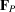denote the contribution of the P atoms to the structure, andand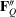the real and imaginary components of the contribution of the Q atoms. The relation between the different contributions to a reflection h and its Friedel equivalentis illustrated in Fig. 2.4.3.2. For simplicity we assume here that all Q atoms are of the same type. The phase angle ofis then exactlyahead of that of. The structure factors of h andare denoted in the figure by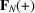and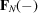, respectively. In the absence of anomalous scattering, or when the imaginary component of the dispersion correction is zero, the magnitudes of the two structure factors are equal and Friedel's law is obeyed; the phase angles have equal magnitudes, but opposite signs. As can be seen from Fig. 2.4.3.2, this is no longer true whenhas a nonzero value. Friedel's law is then violated. A composite view of the vector relationship for h andcan be obtained, as in Fig. 2.4.3.3, by reflecting the vectors corresponding toabout the real axis of the vector diagram.andcorresponding to the two reflections superpose exactly, butdo not.andthen have different magnitudes and phases.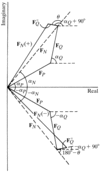Figure 2.4.3.2 | top | pdf |Vector diagram illustrating the violation of Friedel's law when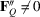.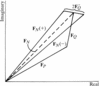Figure 2.4.3.3 | top | pdf |A composite view of the vector relationship betweenand.

It is easily seen that Friedel's law is obeyed in centric data even when anomalous scatterers are present.andare then parallel to the real axis andperpendicular to it. The vector sum of the three components is the same for h and. It may, however, be noted that the phase angle of the structure factor is then no longer 0 or. Even when the structure is noncentrosymmetric, the effect of anomalous scattering in terms of intensity differences between Friedel equivalents varies from reflection to reflection. The difference betweenandis zero when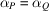or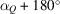. The difference tends to the maximum possible value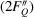when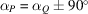.

Intensity differences between Friedel equivalents depend also on the ratio (in terms of number and scattering power) between anomalous and normal scatterers. Differences obviously do not occur when all the atoms are normal scatterers. On the other hand, a structure containing only anomalous scatterers of the same type also does not give rise to intensity differences. Expressions for intensity differences between Friedel equivalents have been derived by Zachariasen (1965) for the most general case of a structure containing normal as well as different types of anomalous scatterers. Statistical distributions of such differences under various conditions have also been derived (Parthasarathy & Srinivasan, 1964; Parthasarathy, 1967). It turns out that, with a single type of anomalous scatterer in the structure, the ratio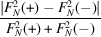has a maximum mean value when the scattering powers of the anomalous scatterers and the normal scatterers are nearly the same (Srinivasan, 1972). Also, for a given ratio between the scattering powers, the smaller the number of anomalous scatterers, the higher is the mean ratio.

### ReferencesParthasarathy, S. (1967). Expectation value of the Bijvoet ratio. Acta Cryst. 22, 98–103.Google ScholarParthasarathy, S. & Srinivasan, R. (1964). The probability distribution of Bijvoet differences. Acta Cryst. 17, 1400–1407.Google ScholarSrinivasan, R. (1972). Applications of X-ray anomalous scattering in structural studies. In Advances in structure research by diffraction methods, Vol. 4, edited by W. Hoppe & R. Mason, pp. 105–197. Braunschweig: Freidr. Vieweg & Sohn; and Oxford: Pergamon Press.Google ScholarZachariasen, W. H. (1965). Dispersion in quartz. Acta Cryst. 18, 714–716.Google Scholar